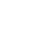# Use Conditional Statements in Bash

Published 3 years ago
Updated 3 years ago

Knowing how conditionals work in bash open up a world of scripting possibilities. We’ll learn the basic syntax, including `if`, `else`, and `elif`. Then we'll look at a few of the "primary" operators you can leverage in a conditional statement such as `=` for string equality, `-eq` for numeric equality, and `-e` to check if a file exists. After that, we'll use conditional statements to create a function that asserts that the HTTP status of a given URL is between 200 and 299.

Instructor: [00:00] For this lesson, I've already created a script file, conditionals.sh, and I've given it xu permissions using the ch mod command.

[00:08] Conditionals in Bash take this basic form. We do if, two square brackets -- here is where our expression goes. I will come back to that -- do semicolon, and then we close it with a fi or a backwards if.

[00:25] Here, we can do all kinds of different checks. Let's check if the user, the global environment variable user, is equal to [inaudible] . If it's true, this is where we can execute whatever we want if this condition is true. I go true. If it's false, we'll just do our else statement there before the closing fi. We'll do [inaudible] false.

[00:46] We run that. We can see that it's true. If we wanted to send and get that up here, we can. Let's run that. That's false. There's a lot of different kinds of expressions that are supported here. Some of the syntax is different from most programing languages.

[01:00] For example, if I wanted to do a numeric equality comparison, if I wanted to see if 1=1, I use this EQ -- these are called primaries. We see that's true, not equal is NE, which is false. There's a lot of different primaries. Some are uniquely suited to Bash.

[01:22] For example, to check if a file exists, you do E, and then we'll check if our scripts here exist, which it should. We see that's true. Another one we can do is Z, which checks if a variable is empty. If I check the user variable and run that, it should be false because it is set.

[01:41] Note that if everyone is an else/if statement, we do that using the LF keyword and then we put our conditional statement in here. Then then, and then this is where we execute code if that conditional is true. I'll just do LF true. Let's run that. Cool, it works.

[02:00] Also, note that this conditional block can be used inline in a more ternary form. To see that in action, we could go up here. We'll say if user equal, we're going to do the double ampersand to say if that's true, and then we can do with the double pipe, just like most programing languages, to add a false case to that. Do that again. We can see that it's coming in there as yes.

[02:25] Let's use conditionals in a more realistic scenario. Let's write a function that curls a URL and checks if that URL is responding with the 200 range status. Call that function, check status.

[02:40] The first thing we need to do is we'll create a local variable called status. We will set up a curl command in here that returns just the HTTP code of that curl.

[02:50] To figure how to do that, it's helpful to jump over to the command line really fast. Let's do curl. Let's use some flags. We're going to do the include headers flag. This will include all the response headers and not the actual response of the URL, because we just want the status header.

[03:07] We'll pass the L, the capital L, which tells it to follow redirects. Then we'll tell it to be silent so that we're not getting progress bars and all that stuff that muddies up the output. Then we'll pass the URL. We'll do sample.org.

[03:22] We see we're getting the response headers. What we specifically want is on this first row with the 200 here. Let's do it again. We'll pipe it to the head commands, and we'll tell it to return just that first row.

[03:36] Now we need to tell it we want it to cut out all of this part and just give the 200. To do that, we use the cut commands. We pass the D flag. It's just for delimiter. We're going to tell it to cut on empty spaces. It's going to split it right there.

[03:54] Then we're going to tell it we want the second part. Refer to cut and heads documentation to see all the different possibilities here. If we run that, we see that it's working.

[04:04] This is the command we want to plug in over here. Now we want to do our if check. We're going to say if the status is less than 200 or greater than 299, we're going to do or. This is our fail case. That's [inaudible] statement explaining what happened.

[04:25] Else, it's succeeded. Let's do the same statement. I want it to say succeeded. Cool. Then up here, we'll return our [inaudible] status.

[04:36] Let's go ahead and try this out. We'll call example.org again. That one should work. Let's call a URL that we know will fail. Let's check that and make sure it worked. Yes, it looks like it's working.Daniel Ram
~ 3 years ago

This may help others: https://stackoverflow.com/questions/18928260/if-command-with-user-input-osx-terminal